# Transfer Functions of Cascaded Circuits

   

The general transfer function of two cascaded circuits is presented and its application to different circuits is explained with examples and their solutions.
Problems and their solutions are also included. Problems and their solutions are also included.

## A - Transfer Function of Two Cascaded Circuits Formula

We consider the two cascaded circuits shown below and find the transfer function $$\dfrac{V_{out}}{V_{in}}$$ in terms of the four $$Z_1, Z_2, Z_3$$ and $$Z_4$$

We use Kirchhoff's current and voltage and Ohm's law to write the equations
$$\qquad I = I_1 + I_2 \qquad (I)$$   Kirchhoff's current law at upper node
$$\qquad V_{in} = Z_1 I + Z_2 I_2 \qquad (II)$$   Kirchhoff's voltage law on closed loop on the left
$$\qquad Z_2 I_2 = (Z_3 + Z_4) I_1 \qquad (III)$$   Kirchhoff's voltage law on closed loop on the right
$$\qquad V_{out} = Z_4 I_1 \qquad (IV)$$   Ohm's law for voltage across $$R_2$$
Use equations (II) and (IV) to write the transfer function $$H( s )$$ as follows
$$\qquad H(s) = \dfrac{V_{out}}{V_{in}} = \dfrac{ Z_4 I_1 }{Z_1 I + Z_2 I_2}$$
Use equation (I) to substitute $$I$$ by $$I_1 + I_2$$ in $$H(s)$$ above
$$\qquad H(s) = \dfrac{ Z_4 I_1 }{Z_1 ( I_1 + I_2) + Z_2 I_2}$$
Divide the numerator and denominator of the above by $$I_1$$, simplify and rewrite as
$$\qquad H(s) = \dfrac{Z_4}{Z_1 \left( 1+ \dfrac{I_2}{I_1} \right) + Z_2 \dfrac{I_2}{I_1}} \qquad (V)$$
Use equation (III) to obtain
$$\qquad \dfrac{I_2}{I_1} = \dfrac{Z_3 + Z_4}{Z_2}$$

Substitute $$\dfrac{I_2}{I_1}$$ by the above in $$(IV)$$ and rearrange to obtain $$H(s)$$

$H(s) = \dfrac{Z_4 Z_2 }{(Z_1 + Z_2)(Z_4 + Z_3 ) + Z_1 Z_2} \qquad (I)$

## B - Application of the Transfer Function of Two Cascaded Circuits Formula

It is now shown how the above formula could be used in any circuit that may be identified as a two-cascased circuits.
Example 1
Find the transfer function in the frequency domain of the circuit below.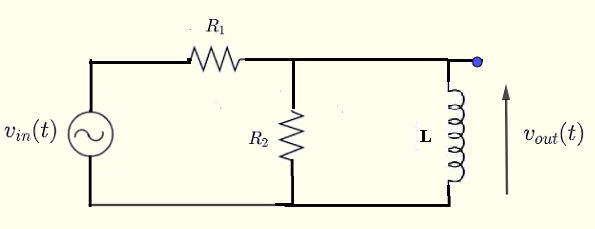Solution to Example 1
Comparing the given circuit with the more general circuit above, we can write
$$\qquad Z_1 = R_1$$ , $$Z_2 = R_2$$ , $$Z_3 = 0$$ and $$Z_4 = L s$$
where $$s = j \omega$$ and $$\omega$$ is the angular frequency.
We now substitute the impedances $$Z_1, Z_2, Z_3$$ and $$Z_4$$ in the general formula obtained above to write
$$\qquad H(s) = \dfrac{R_2 \; L \; s }{(R_1 + R_2) \; L\;s + R_1 \; R_2}$$
Substitute $$s = j \omega$$ and write
$$\qquad H(\omega) = \dfrac{j \; R_2 \; L \; \omega\; s }{j \; (R_1 + R_2) \; \omega \; L\;s + R_1 \; R_2}$$

Example 2
Find the transfer function in the frequency domain of the circuit below.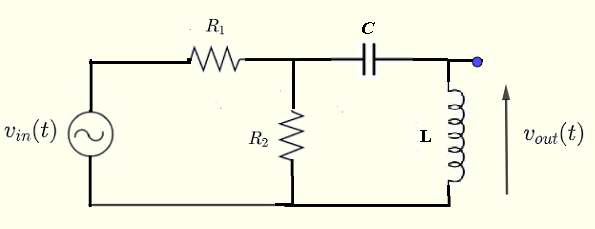Solution to Example 2
Comparing the given circuit with the more general circuit above, we can write
$$\qquad Z_1 = R_1$$ , $$Z_2 = R_2$$ , $$Z_3 = \dfrac{1}{C s}$$ and $$Z_4 = L s$$
where $$s = j \omega$$ and $$\omega$$ is the angular frequency.
We now substitute the impedances $$Z_1, Z_2, Z_3$$ and $$Z_4$$ in the general formula obtained above to write
$$\qquad H(s) = \dfrac{R_2 \; L \; s }{(R_1 + R_2) (\; L\;s + \dfrac{1}{C s}) + R_1 \; R_2}$$
Mutliply numerator and denominator by $$C s$$ and simplify
$$\qquad H(s) = \dfrac{R_2 \; L \; C \; s^2 }{(R_1 + R_2) C \; L\;s^2 + R_1 \; R_2 \; C s + R_1 + R_2}$$
Substitute $$s = j \omega$$ and write
$$\qquad H(\omega) = \dfrac{- R_2 \; L \; C \; \omega^2 }{ - (R_1 + R_2) C \; L\;\omega^2 + j \; R_1 \; R_2 \; C \omega + R_1 + R_2}$$

Example 3
Find the transfer function in the frequency domain of the circuit below and graph it magnitude and argument (or phase).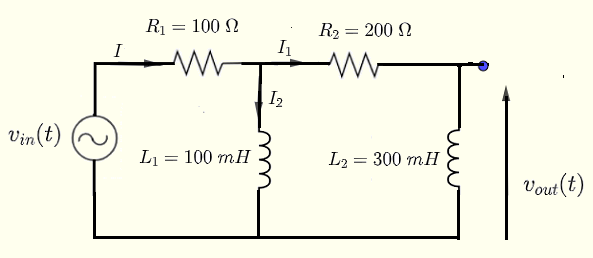Solution to Example 3

Use the formula in (I) above
$$\qquad H(s) = \dfrac{Z_4 Z_2 }{(Z_1 + Z_2)(Z_4 + Z_3 ) + Z_1 Z_2}$$

We now calculate the impedances $$Z_1, Z_2, Z_3$$ and $$Z_4$$ using the given numerical values in the circuit in part b).
$$\qquad Z_1 = 100$$ , $$\qquad Z_2 = 0.1 s$$ , $$Z_3 = 200$$ , $$Z_4 = 0.3 s$$
We now substitute to obtain
$$\qquad H(s) = \dfrac{0.03 \; s^2}{(100 + 0.1 \; s)(0.3 s + 200 ) + 10 \; s}$$
Simplify
$$\qquad H(s) = \dfrac{3 \; s^2}{3 \; s^2 + 6000 \; s + 2000000}$$
Substitute $$s$$ by $$j\; \omega$$
$$\qquad H(\omega) = \dfrac{ - \; 3 \; \omega^2}{- 3 \omega^2 + 2000000+ j \; 6000 \; \omega }$$

## Problems with Solutions

Find the transfer function in the frequency domain for each circuit below in parts A and B.
Part A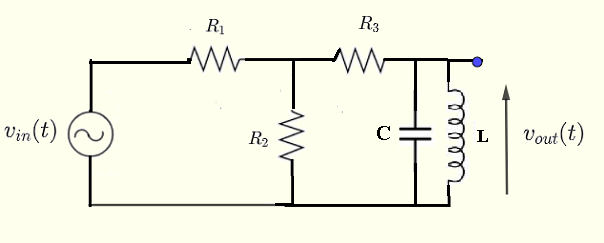Part B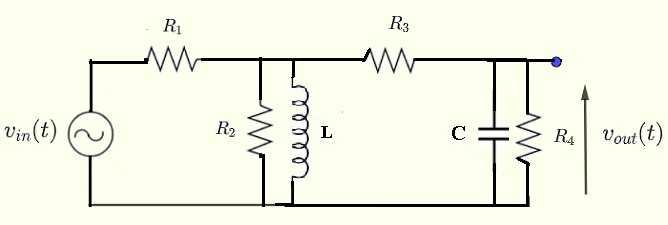## Solutions to the Above Problems

Part A
Let $$\qquad s = j \; \omega$$, $$Z_1 = R_1$$ , $$Z_2 = R_2$$, $$Z_3 = R_3$$ and $$Z_4 = C // L = \dfrac{Ls}{C L s^2 + 1}$$
Substitute $$Z_1, Z_2, Z_3$$ and $$Z_4$$ by their expressions in formula (I) above
$$\qquad H(s) = \dfrac{ \dfrac{Ls}{C L s^2 + 1} R_2 }{(R_1 + R_2)\left( \dfrac{Ls}{C L s^2 + 1} + R_3 \right) + R_1 R_2}$$

Multiply numerator and denominator by $$(C L s^2 + 1)$$ and simplify

$$\qquad H(s) = \dfrac{ LR_2 s }{(R_1 + R_2)\left( L s + R_3 (C L s^2 + 1) \right) + R_1 R_2 (C L s^2 + 1)}$$
Expand and factor expressions in the denominator
$$\qquad H(s) = \dfrac{ LR_2 s }{ CL(R_1 R_3 + R_2R_3 + R_1 R_2)s^2 + L(R_1+R_2)s + R_1 R_3 + R_2 R_3 + R_1 R_2}$$
Substitute $$s$$ by $$j \omega$$ to obtain
$$\qquad H(\omega) = \dfrac{ j \; LR_2 \; \omega }{ -CL(R_1 R_3 + R_2R_3 + R_1 R_2) \; \omega^2 + j \; L(R_1+R_2) \; \omega + R_1 R_3 + R_2 R_3 + R_1 R_2}$$

Part B
Let $$\qquad s = j \omega$$, $$Z_1 = R_1$$ , $$Z_2 = R_2 // L = \dfrac{R_2 L s}{L s + R_2}$$, $$Z_3 = R_3$$ and $$Z_4 = C // R_4 = \dfrac{R_4}{1+R_4 C s}$$
Substitute $$Z_1, Z_2, Z_3$$ and $$Z_4$$ by their expressions in formula (I) above

$$\qquad H(s) = \dfrac{ \left(\dfrac{R_4}{1+R_4 C s} \right) \left(\dfrac{R_2 L s}{L s + R_2} \right) }{ \left(R_1 + \dfrac{R_2 L s}{L s + R_2} \right) \left(\dfrac{R_4}{1+R_4 C s} + R_3 \right) + R_1 \dfrac{R_2 L s}{L s + R_2}}$$

Multiply the numerator and the denominator by the expression $$(1+R_4 C s) (L s + R_2)$$ and simplify

$$\qquad H(s) = \dfrac{R_4R_2Ls}{ (R_1 (L s + R_2) + R_2 Ls) (R_4 + R_3 (1+R_4 C s)) + R_1 R_2 Ls (1+R_4 C s) }$$
Expand expressions in the denominator
$$\qquad H(s) = \dfrac{R_4R_2Ls}{LsR_2R_1+LR_4Cs^2R_2R_1+LsR_1R_3+LR_4Cs^2R_1R_3+LR_4sR_1+R_2R_1R_3+R_4CsR_2R_1R_3+R_4R_2R_1+LsR_2R_3+LR_4Cs^2R_2R_3+LR_4sR_2}$$
Group terms with $$s^2$$ and terms with $$s$$ and factor
$$\qquad H(s) = \dfrac{R_4R_2Ls}{ (LR_4CR_2R_1+ LR_4CR_1R_3+ LR_4CR_2R_3 )s^2 + (LR_2R_1+LR_1R_3+LR_4R_1+R_4CR_2R_1R_3+LR_2R_3+LR_4R_2)s +R_2R_1R_3 +R_4R_2R_1 }$$
Substitute $$s = j \omega$$ and write
$$\qquad H(\omega) = \dfrac{j \; R_4R_2L \; \omega}{ - (LR_4CR_2R_1+ LR_4CR_1R_3+ LR_4CR_2R_3 ) \; \omega^2 + j \; (LR_2R_1+LR_1R_3+LR_4R_1+R_4CR_2R_1R_3+LR_2R_3+LR_4R_2) \; \omega +R_2R_1 (R_3 +R_4) }$$